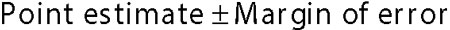##### Statistics for Big Data For DummiesAlthough EDA is mainly based on graphical techniques, it also consists of a few quantitative techniques. This article discusses two of these: interval estimation and hypothesis testing.

## Interval estimation

Interval estimation is a technique that's used to construct a range of values within which a variable is likely to fall. One important example of this is the confidence interval. A confidence interval is a range of numbers that is likely to contain the value of a population measure such as the mean. A confidence interval is constructed as follows:The confidence interval consists of a lower limit equal to the point estimate minus the margin of error, and an upper limit equal to the point estimate plus the margin of error.

The point estimate is a single value estimated from a sample. For example, the sample mean is a point estimate of the population mean. Similarly, the sample standard deviation is a point estimate of the population standard deviation.

The margin of error reflects the amount of uncertainty associated with the point estimate. In other words, it shows how much the point estimate can change from one sample to the next. The margin of error is based on the standard deviation and the size of the sample being used. The result of these calculations is a range of values that is likely to contain the true value of the population measure.

For example, suppose a researcher determines that with 95 percent confidence, the interval (–2.0 percent, +8.0 percent) contains the true value of the mean return to the S&P 500 next year. The sample mean is the average of the lower and upper limit of this interval (that is, 3.0 percent). The margin of error is therefore 5 percent.

## Hypothesis testing

A statistical hypothesis is a statement that's assumed to be true unless there's strong contradictory evidence. Hypothesis testing is widely used in many disciplines to determine whether a proposition is true or false. For example, hypothesis testing could be used to determine whether

• The mean age of the residents of a state is 43 years old.

• The mean return to the stocks in a portfolio is 7.2 percent.

• The amount of annual rainfall in a city follows the normal distribution.

Hypothesis testing is a multi-step process consisting of the following:

1. The statement of the null hypothesis: This is the statement that is assumed to be true.

2. The statement of the alternative hypothesis: This is the statement that will be accepted if the null hypothesis is rejected.

3. The level of significance at which the hypothesis test will be conducted: This equals the likelihood of rejecting the null hypothesis when it is false.

4. The test statistic: This is a numerical measure that shows whether sample data is consistent with the null hypothesis.

5. The critical value: If the test statistic is more extreme than the critical value, the null hypothesis is rejected.

6. The decision: Based on the relationship between the test statistic and the critical value, you make a decision as to whether or not the null hypothesis should be rejected.